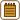## Heating of water in electric kettle

We poured 1.2 l of water at 15 °C in a 2000 W kettle. When the water started to boil, the kettle turned off.

a) How long would the heating take in the ideal case?

b) In fact, the heating took about 30 s longer. What does that imply?

• #### Notation

 V = 1.2 l = 1.2·10−3 m3 Water volume ρ = 1000 kg·m3 Water density TB = 100 °C Boiling point of water T = 15 °C Initial temperature of water P = 2000 W Power of the kettle Δτ = 30 s Difference between the actual and the assumed heating times τ = ? Heating time of water η = ? Kettle efficiency

From the tables:

 cW = 4180 J kg−1K−1 Specific heat capacity of water
• #### Hint a)

What is the relationship between the kettle power, the heat it delivers and time?

• #### Analysis a)

We need to add heat to the water to heat it up from the initial temperature to the boiling point. The kettle performance tells us how much heat the kettle delivers to the water in 1 second. We can then determine the heating time from the known power and the heat required.

• #### Solution a)

First, we calculate the heat needed to make the water start boiling (the heat we need to heat it up to TB = 100 °C).

The delivered heat Q, is given by the relationship:

$Q=m \cdot c_{\mathrm{W}}(T_{\mathrm{B}}-T)=V\cdot\rho\cdot c_{\mathrm{W}}(T_{\mathrm{B}}-T)\,,$

where m is the weight of water that we warm from the initial temperature T to the boiling point TB and cW is the specific heat capacity of the water. We expressed the weight of water using its volume V and density ρ.

The time τ needed for heating is then obtained as the ratio of the supplied heat Q and the power P:

$\tau=\frac{Q}{P}=\frac{V \cdot \rho \cdot c_{\mathrm{W}} (T_{\mathrm{B}}-T)}{P}\,.$

Numerical substitution:

$\tau=\frac{V \cdot \rho \cdot c_{\mathrm{W}} (T_{\mathrm{B}}-T)}{P}=\frac{1.2\cdot{10^{-3}}\cdot{1000}\cdot{4180}\cdot{(100-15)}}{2000}\,\mathrm s \,\dot=\, 213\,\mathrm s\,.$
• #### Hint b)

What means the efficiency of the appliance and why is it usually less than 100%?

• #### Analysis b)

The fact that the heating in reality took longer indicates that the efficiency of the kettle is less than 100%, since it also heats the material from which the kettle is made and a part of the heat leaks to the surroundings. An important role is also played by the fact that the water is evaporating after reaching the boiling point and takes a part of the energy for that.

The efficiency can be calculated as the ratio of energy used to heat water and the total energy delivered. As will be deduced in the Solution section, the efficiency is also equal to the ratio of the expected and the actual heating time.

• #### Solution b)

The fact that the heating actually took longer is due to the limited efficiency of the appliance. The efficiency is defined as the ratio of the delivered power P and the input power P0

$\eta =\frac{P}{P_0}\,,$

that is, the ratio of energy consumed for heating the water and the energy consumed by the kettle.

The energy consumed to heat the water can be expressed as the product of the kettle power consumption P0 and the time τ calculated in task a). (This gives the energy that would be transmitted at 100% efficiency of the kettle).

The energy actually consumed by the kettle is expressed as the product of the input power P0 and the actual time that the water heating took τ + Δτ.

So we can write:

$\eta=\frac{P_0\tau}{P_0\left(\tau+\mathrm{\Delta}\tau\right)}\,.$

After cancelling P0 out, we get the relationship:

$\eta =\frac{\tau}{\tau+\mathrm{\Delta}\tau}\,,$

into which we can already substitute.

Numerical substitution:

$\eta =\frac{\tau}{\tau+\mathrm{\Delta}\tau}=\frac{213}{213+30}\,\dot= 0.877=87.7\%$

a) At 100% efficiency of the kettle, the heating would take approximately 213 seconds.

b) The actual heating time is longer due to the limited efficiency of the appliance, which is about 87.7% in this case.

• #### A matter of interest

The efficiency of the kettle was almost 88% in this task. Manufacturers report that the efficiency of the kettle can reach up to 90%. It depends on the type of kettle and in most cases it will probably be a bit smaller.

It also plays a big role in the value of efficiency whether we heat a full or a half-empty kettle. The fuller the kettle, the higher the efficiency of the water heating.

• #### Link to an experiment

An experiment with a similar topic can be found here: Experimental determination of Specific Heat of Water.